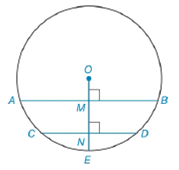Chapter 6.4, Problem 37E### Elementary Geometry for College St...

6th Edition
Daniel C. Alexander + 1 other
ISBN: 9781285195698

#### Solutions

Chapter
Section### Elementary Geometry for College St...

6th Edition
Daniel C. Alexander + 1 other
ISBN: 9781285195698
Textbook Problem
36 views

# In ⊙ O , chord A B ¯ ∥ chord C D ¯ . Radius O E ¯ is perpendicular to A B ¯ and C D ¯ at points M and N , respectively. If O E = 13 , A B = 24 , and C D = 10 , then the distance from O to C D ¯ is greater than the distance from O to A B ¯ . Determine how much farther chord C D ¯ is from center O than chord A B ¯ is from center O ; that is, find M N .To determine

To find:

The distance chord CD¯ is farther from center O than chord AB¯ is from center O; that is, find MN.

Explanation

Given:

O, chord AB¯ chord CD¯. Radius OE¯ is perpendicular to AB¯ and CD¯ at points M and N, respectively. OE=13, AB=24, and CD=10, such that the distance from O to CD¯ is greater than the distance from O to AB¯.

The figure given below,

Theorem Used:

According to the Pythagorean theorem, in a right-angled triangle,

hypotenuse2=base2+perpendicular2.

Calculation:

Since the chords AB=24, and CD=10 are unequal i.e. mCD¯>mAB¯ and the distance from O to CD¯ is greater than the distance from O to AB¯ i.e. mON¯>mOM¯ and applying Pythagorean theorem from the figure below,

In AOB,

OB¯2=OM¯2+MB¯2 or OM¯=OB¯2MB¯2

And from COD

OC¯2=ON¯2+NC¯2 or ON¯=OC¯2NC¯2

Since OM and ON are perpendicular bisector as a radius perpendicular to a chord bisects the chord and its arc

### Still sussing out bartleby?

Check out a sample textbook solution.

See a sample solution

#### The Solution to Your Study Problems

Bartleby provides explanations to thousands of textbook problems written by our experts, many with advanced degrees!

Get Started

#### Find for the functions in Problems 7-10. 8.

Mathematical Applications for the Management, Life, and Social Sciences

#### In Exercises 6372, evaluate the expression. 64. 4 + |4|

Applied Calculus for the Managerial, Life, and Social Sciences: A Brief Approach

#### Determine whether f'(0) exists. f(x)={x2sin1xifx00ifx=0

Single Variable Calculus: Early Transcendentals, Volume I

#### The arc length of y = 3x + 2 from x = 1 to x = 5 is a) 210 b) 52 c) 102 d) 410

Study Guide for Stewart's Single Variable Calculus: Early Transcendentals, 8th